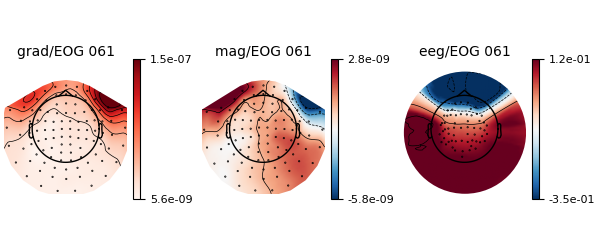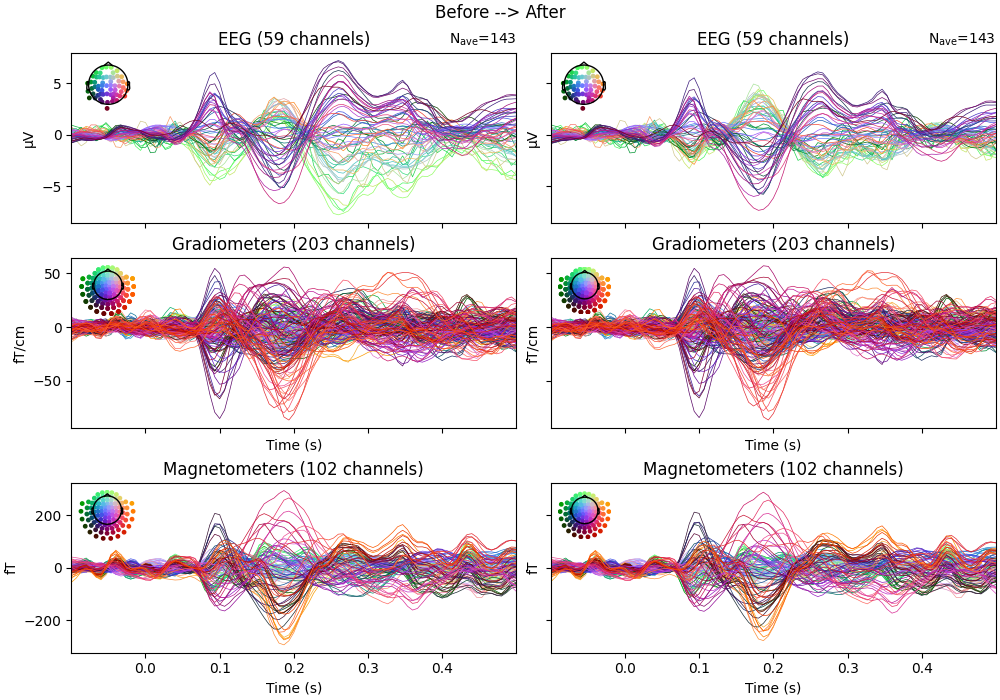# Reduce EOG artifacts through regression#

Reduce artifacts by regressing the EOG channels onto the rest of the channels and then subtracting the EOG signal.

This is a quick example to show the most basic application of the technique. See the tutorial for a more thorough explanation that demonstrates more advanced approaches.

```# Author: Marijn van Vliet <w.m.vanvliet@gmail.com>
#
```

## Import packages and load data#

We begin as always by importing the necessary Python modules and loading some data, in this case the MNE sample dataset.

```import mne
from mne.datasets import sample
from mne.preprocessing import EOGRegression
from matplotlib import pyplot as plt

print(__doc__)

data_path = sample.data_path()
raw_fname = data_path / "MEG" / "sample" / "sample_audvis_filt-0-40_raw.fif"

events = mne.find_events(raw, "STI 014")

# Highpass filter to eliminate slow drifts
raw.filter(0.3, None, picks="all")
```
```Opening raw data file /home/circleci/mne_data/MNE-sample-data/MEG/sample/sample_audvis_filt-0-40_raw.fif...
Read a total of 4 projection items:
PCA-v1 (1 x 102)  idle
PCA-v2 (1 x 102)  idle
PCA-v3 (1 x 102)  idle
Average EEG reference (1 x 60)  idle
Range : 6450 ... 48149 =     42.956 ...   320.665 secs
Reading 0 ... 41699  =      0.000 ...   277.709 secs...
319 events found
Event IDs: [ 1  2  3  4  5 32]
Filtering raw data in 1 contiguous segment
Setting up high-pass filter at 0.3 Hz

FIR filter parameters
---------------------
Designing a one-pass, zero-phase, non-causal highpass filter:
- Windowed time-domain design (firwin) method
- Hamming window with 0.0194 passband ripple and 53 dB stopband attenuation
- Lower passband edge: 0.30
- Lower transition bandwidth: 0.30 Hz (-6 dB cutoff frequency: 0.15 Hz)
- Filter length: 1653 samples (11.009 s)

[Parallel(n_jobs=1)]: Done  17 tasks      | elapsed:    0.0s
[Parallel(n_jobs=1)]: Done  71 tasks      | elapsed:    0.2s
[Parallel(n_jobs=1)]: Done 161 tasks      | elapsed:    0.3s
[Parallel(n_jobs=1)]: Done 287 tasks      | elapsed:    0.6s
```
Measurement date December 03, 2002 19:01:10 GMT Unknown Unknown 146 points 204 Gradiometers, 102 Magnetometers, 9 Stimulus, 60 EEG, 1 EOG MEG 2443, EEG 053 EOG 061 Not available 150.15 Hz 0.30 Hz 40.00 Hz PCA-v1 : offPCA-v2 : offPCA-v3 : offAverage EEG reference : off sample_audvis_filt-0-40_raw.fif 00:04:38 (HH:MM:SS)

## Perform regression and remove EOG#

```# Fit the regression model
weights = EOGRegression().fit(raw)
raw_clean = weights.apply(raw, copy=True)

# Show the filter weights in a topomap
weights.plot()
``````Created an SSP operator (subspace dimension = 4)
4 projection items activated
SSP projectors applied...
```

## Before/after comparison#

Let’s compare the signal before and after cleaning with EOG regression. This is best visualized by extracting epochs and plotting the evoked potential.

```tmin, tmax = -0.1, 0.5
event_id = {"visual/left": 3, "visual/right": 4}
evoked_before = mne.Epochs(
raw, events, event_id, tmin, tmax, baseline=(tmin, 0)
).average()
evoked_after = mne.Epochs(
raw_clean, events, event_id, tmin, tmax, baseline=(tmin, 0)
).average()

# Create epochs after EOG correction
epochs_after = mne.Epochs(raw_clean, events, event_id, tmin, tmax, baseline=(tmin, 0))
evoked_after = epochs_after.average()

fig, ax = plt.subplots(nrows=3, ncols=2, figsize=(10, 7), sharex=True, sharey="row")
evoked_before.plot(axes=ax[:, 0], spatial_colors=True)
evoked_after.plot(axes=ax[:, 1], spatial_colors=True)
top=0.905, bottom=0.09, left=0.08, right=0.975, hspace=0.325, wspace=0.145
)
fig.suptitle("Before --> After")
``````Not setting metadata
143 matching events found
Applying baseline correction (mode: mean)
Created an SSP operator (subspace dimension = 4)
4 projection items activated
NOTE: pick_channels() is a legacy function. New code should use inst.pick(...).
143 matching events found
Applying baseline correction (mode: mean)
Created an SSP operator (subspace dimension = 4)
4 projection items activated
NOTE: pick_channels() is a legacy function. New code should use inst.pick(...).